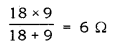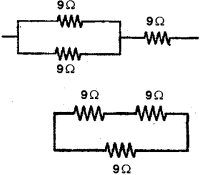# Show how could you join three resistors, each of resistance 9 Ω

Show how could you join three resistors, each of resistance 9 Ω so that the equivalent resistance of the combination is
(i) 13.5 Ω
(ii) 6 Ωtwo 9 n resistors are connected in parallel and one in sensor

(ii) 2 resistors connected in series = (9 + 9) Ω = 18Ω
18 Ω and 9 Ω are connected in series.